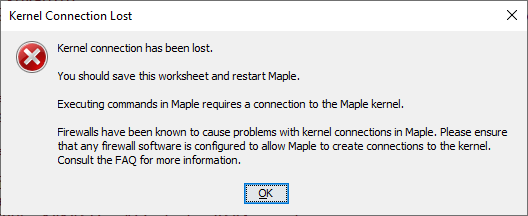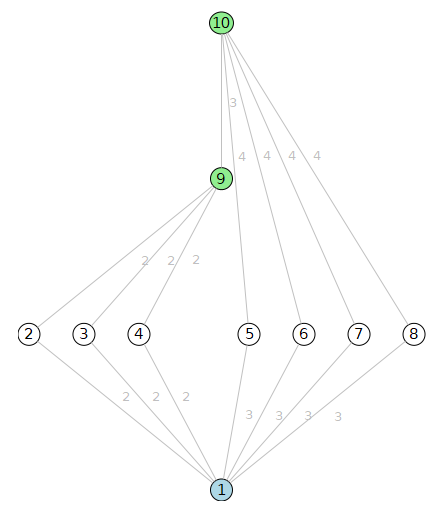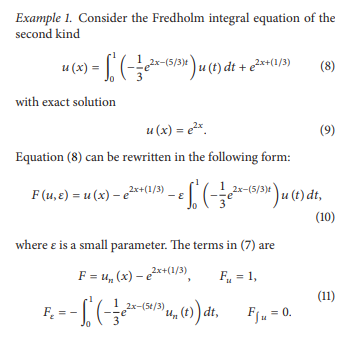## Solve ODE in fourier space numerically and transfo...

Dear all,

consider two lists of complex values :

list1 := [l1,l2,l3,l4,l5]

list2 := [s1,s2,s3,s4,s5].

There is a set of second order differential equation

d^2u(k)/dt^2+I*A*du/dt-B*u=0

where A is sum of elements of list1 and list2 and B is multiplication of their element. Therefore,

d^2u(k)/dt^2+I*(l1+s1)*du/dt-(l1*s1)*u=0

d^2u(k)/dt^2+I*(l2+s2)*du/dt-(l2*s2)*u=0

d^2u(k)/dt^2+I*(l3+s3)*du/dt-(l3*s3)*u=0

d^2u(k)/dt^2+I*(l4+s4)*du/dt-(l4*s4)*u=0

d^2u(k)/dt^2+I*(l5+s5)*du/dt-(l5*s5)*u=0

How can I create a set of differential equations and initial conditions based on nops(list1), then solve this system of differential equations numerically in Maple.

since u[i] are function of k, next step is to transforme them to real space by inverse fourier transform.

finally save the results and plot them.

Note that for simplisity I wrote a linear equation but it is not. so, because of nonlinear terms it is not possible to use superposition of the solution. I have to take them as coupled system of equations.

====

for example

```list1 := [ [0., -5.496799068*10^(-15)-0.*I], [.1, 5.201897725*10^(-16)-1.188994754*I], [.2, 6.924043163*10^(-17)-4.747763855*I], [.3, 2.297497722*10^(-17)-10.66272177*I], [.4, 1.159126178*10^(-17)-18.96299588*I] ]

list2 :=[ [0., -8.634351786*10^(-7)-67.81404036*I], [.1, -0.7387644021e-5-67.76491234*I], [.2, -0.1433025271e-4-67.59922295*I], [.3, -0.2231598645e-4-67.25152449*I], [.4, -0.3280855430e-4-66.56357035*I] ]```

where first element is k and the second value is l_i and s_i

the differential equation is

`ode_u[i]:= diff(u[i](t),t\$2)+I*(list1[i]+list2[i])*diff(u[i](t),t)-list1*list2*u[i](t)=0;`

eta is in fourier space where k values are in list1[i].

We laso know that f(-k)= - f*(k) where f=list[i]

and u[i] as function of k, initially has a Gaussian shape at t=0 in fourier space..

## nyqlog for Maple...

Anyone out there converted the  nyqlog at MATLAB/nyqlog.m at master · nielsSkov/MATLAB · GitHub  to Maple or Maple Flow? For Nyquist plots...

## Réalisation complète ...

Bonjour, petite question simple que je me pose la MapleSim permet de tout réaliser ?

## why so many "kernel connection has been lost" in M...

I get about 3-4 of these each day when I run my long script. Maple 2022.2 on windows 10They happen much more for me in Maple 2022 than before. I am on windows 10 pro with 128 GB ram, new PC, very fast PC.

googling, I see others having same problem.

"Ever since I upgraded to Maple 2022, relatively intensive symbolic calculations and plots frequently kill the kernel and throw the error message “Kernel Connection has been lost”. This never happened on Maple 2021."

It happens for me whenever I run something that takes long time and it is always random when and where it happens.

This is really disappointing as one expects software quality of a product to improve over time but for me, I find Maple's becoming less robust and its quality becoming worst not better.  This is too bad, because Maple is a good language and I like programming in it, but it is the software build quality of the overall software which needs to be improved.

I see many hangs also where I have to kill mserver.exe may be 10 times a day just to get the script to complete. (this is besides this kernel lost connection problem). Those also happen at random places in the script. Restarting the script from same place, it runs OK now.

Is there something one can do to find why this lost connection happens?  Crash dump somewhere? Log file somewhere?

I do not have firewall. I use default windows 10 settings for security. Do not have any other special anti-virus software.  No other app I use on this PC crash like this. It is only Maple.

## Error in in Importing a Matrix from matlab to mapl...

I want to import a numeric 2800*1 matrix from matlab to maple by following command, but faced error as bellow:

X := ImportMatrix("E:/.../Omega.mat", source = MATLAB);
ImportMatrix:-ModuleApply called with arguments: E:/.../Omega.mat, datatype = auto, delimiter = (), format = (), mode = (), output = all, ragged = true, skiplines = 0, source = MATLAB, sourceid = all, transpose = false
#(ImportMatrix:-ModuleApply,36): error
Error, (in ImportMatrix) Array index out of range
locals defined as: file = E:/.../Omega.mat, src = Matlab, ext = ext, res = res, x = x, isv7 = isv7, del = false

Where is the problem?

How to import?

## Solve numerical PDE...

Hello guys
I'm having trouble solving a PDE using pdsolve-numerical. Here's a notebook attached.

I'm grateful if anyone can help.

Regards,

Oliveira

## How to solve PDE with regular derivatives as coeff...

I would appreciate any help to solve the following Partial Differential Equation, which is a mix of partial and regular derivatives as coefficients.

How to solve it with/without initial conditions?## How to read smiles file using quantum chemistry to...

How to read the topological structure of chemical from a SMILES file in Maple

As I want only the physical structure of the molecule

Like in molecular data it gives the bonds of topology {1,2} like the structure it gives

As SMILES can give 2D structure to me

Kind help how to do

## Variational formulation of GR...

I am new to both the variational formulation of GR and Maple. Attached is the worksheet I am working on. I am wanting to calculate the variation with respect of u and the metric tensor.

## In Maple, how do I show two geometric regions (def...

Let us begin with few simulations:

 > restart;
 >memory used=0.57TiB, alloc change=91.51MiB, cpu time=18.77m, real time=15.86m, gc time=4.91m>memory used=0.56TiB, alloc change=-12.08MiB, cpu time=18.70m, real time=15.11m, gc time=5.69mWell, I'd like to prove (through the use of Maple):

`transform((x1, x2, x3, x4, x5) -> [x1 - x3, x2 - x4, x5])(inequal(And((x || (1 .. 5)) >=~ 0, norm([x || (1 .. 5)], 1) = 1))) # not Maple syntax`

is equivalent to a filled pyramid

`ImplicitRegion((X, Y, Z), 0 <= Z <= 1 - abs(X) - abs(Y)) # not SymPy syntax`

`transform((x1, x2, x3, x4, x5) -> [x1 - x3, x2 - x4, x5])(inequal(And((x || (1 .. 5)) >=~ 0, norm([x || (1 .. 5)], 2) = 1))) # not Maple syntax`

is equivalent to a hemi-ball

`ImplicitRegion((X, Y, Z), 0 <= Z <= sqrt(1 - X**2 - Y**2)) # not SymPy syntax`

, and

`transform((x1, x2, x3, x4, x5) -> [x1 - x3, x2 - x4, x5])(inequal(And((x || (1 .. 5)) >=~ 0, norm([x || (1 .. 5)], 'infinity') = 1))) # not Maple syntax`

is equivalent to a solid cuboid

`ImplicitRegion((X, Y, Z), -1 <= X <= 1 & -1 <= Y <= 1 & 0 <= Z <= 1) # not SymPy syntax`

. (Here, for the convenience of the descriptions, I utilize some non-standard notation from .)
Note that ”two regions are equal" is a two-way property, which means the following proof

```is(Z >= 0) and is(Z <= 1 - abs(X) - abs(Y)) assuming (X, Y, Z) =~ (x1 - x3, x2 - x4, x5), x || (1 .. 5) >=~ 0, add(x || (1 .. 5)) = 1;
true
(*Accordingly, the latter region is a subset of the former one.*) ```

is incomplete (because it's hard to determine whether the is routine always performs equivalent transformations in internal evaluation).

So, can I execute such eliminations in Maple?

## How to calculate the all Intermediate fields of Ga...

As the code:

```poly := x^4 + 8*x + 12:
galois(poly, x)```

"4T4", {"A(4)"}, "+", 12, {"(1 2 4)", "(2 3 4)"}

Then I know it's Galois group has to be (isomorphic to) A4. And I can draw its Subgroup Lattice:

`DrawSubgroupLattice(GaloisGroup(poly, x), 'indices')`But according to Galois's theory, each subgroup represents an intermediate field. As far as I know, ⑤⑥⑦⑧ are Q(r1),Q(r2),Q(r3) and Q(r4), respectively, where ri is the root of equation x^4+8x+12. But I have no idea what fields ②③④⑨ means. How do you calculate out those intermediate fields with maple?

## Parameter Estimation using Nonlinear Least-Squares...

Dear researchers,

Greetings, I have unable to apply Nonlinear Least-Squares Methods  for an SIR parameter estimation. May you share me your Maple code model as a  sample parameter estimation ?

## To plot the primitives of the odometric model, Map...

Dear people,

I have resolved the primitives of the equation of the odometric model.

I have plotted them to the Maple interface and I was thinking that it can have been done by the solver.

Nevertheless it has not been possible to do it.

Can you tell me if a package of Maple can resolve it ?

Best regards,

Edern Ollivier.

## Maple Code to solve Perturbation Iteration Solutio...

Hi,

May  I please get a Maple code to solve the below Perturbation Iteration Solutions for Volterra Integral Equations?

I shall be very much grateful if I can get the Maple Code.

And possible plotting

Thanks## Error message in maple...

 1 2 3 4 5 6 7Last Page 1 of 309